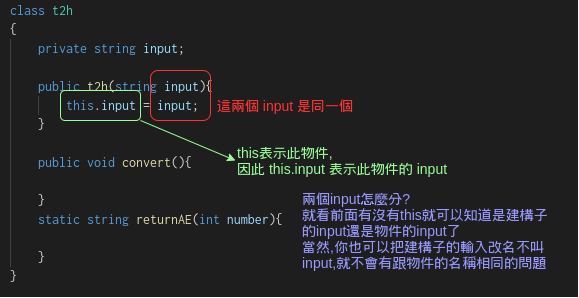#DAY 12
2
Software Development

## 到底什麼是物件?

### 在物件導向的世界裡面,什麼東西都是物件

``````public static int h2t(String input){
int output = 0;
int i = 0;
while (i < input.length() ) {
``````

``````input.length()
``````

``````static void h2t(String input){
double output = 0;
var pow = input.Length;
``````

``````length(input)
``````

``````arrayLength(input)
``````

## 我們來寫自己的物件

``````dotnet new console -n classType
``````

``````using System;

namespace classType  //看到你剛才打的專案名稱了吧
{
class Program
{
static void Main(string[] args)
{
Console.WriteLine("Hello World!");
}
}
}
``````

``````static void Main(string[] args)
{
Console.WriteLine("Tell me what you want to do:");
Console.WriteLine("(1)T to H (2)H to T");
switch (choose)
{
case "1":
t2h.convert();
break;
case "2":
h2t.convert();
break;
default:
Console.WriteLine("Wrong selection");
break;
}
Main();
}
``````

``````var t2h = new t2h(Console.ReadLine());
``````

new是建立物件的關鍵字,這裡建立了一個型別為t2h的物件,初始化的值就是我們的輸入值

t2h的型別? 根本聽都沒聽過阿

``````t2h.cs
``````

(這下你知道為什麼副檔名是cs的原因了吧)

``````namespace classType
{
class t2h
{
private string input;

public t2h(string input){
this.input = input;
}

public void convert(){

}
private string returnAE(int number){

}
}
}
``````

class是物件的關鍵字,使用這個關鍵字可以建立一個物件的藍圖,

### 建構子

``````public t2h(string input){
this.input = input;
}
``````

``````var t2h = new t2h(Console.ReadLine());
````````````public t2h(string input){
this.input = Int32.Parse(input);
}
``````

### 屬性

``````private string input;
``````

private表示我們對外隱藏這個屬性,只有物件本身可以看得到他

``````t2h.input = 120
``````

### 方法

``````t2h.convert()
``````

``````private string returnAE(int number){
``````

``````public void convert(){
try
{
var num = Int32.Parse(input);
for (int i = 0; i < 16; i++)
{
for (int j = 0; j < 16; j++)
{
for (int k = 0; k < 16; k++)
{
if (Math.Pow(16,2)*i+Math.Pow(16,1)*j+Math.Pow(16,0)*k == num)
{
Console.WriteLine("{0}{1}{2}",returnAE(i),returnAE(j),returnAE(k));
}
}
}
}
}
catch (System.Exception)
{
Console.WriteLine("Wrong Input");
}
}
``````

``````public void convert(){
``````

``````var num = Int32.Parse(input); //取用屬性的input
``````

``````public void convert(){
try
{
var input = "12";
var num = Int32.Parse(input); //這時input就會取用方法內部的input而不是較外層的屬性input
for (int i = 0; i < 16; i++)
{
``````

## 物件的使用

``````case "1":
var t2h = new t2h(Console.ReadLine()); //這裡使用了建構子建立物件
t2h.convert();  //這裡取用物件的方法來做使用
break;
case "2":
h2t.convert();
break;
default:
Console.WriteLine("Wrong selection");
break;
``````

``````String number = Console.ReadLine();  //先將讀取到的數值丟數變數內
t2h(number); //再把變數的值丟入方法內
``````

## 同場加映python物件

``````class t2h:
def __init__(self, input):
self.__input = int(input)

def convert(self):
for i in range(16):
for j in range(16):
for k in range(16):
if math.pow(16,2)*i+math.pow(16,1)*j+math.pow(16,0)*k == self.__input:
print(self.__returnAE(i)+self.__returnAE(j)+self.__returnAE(k))

def __returnAE(self, input):
if input<10:
return str(input)
elif input==10:
return "A"
elif input==11:
return "B"
elif input==12:
return "C"
elif input==13:
return "D"
elif input==14:
return "E"
elif input==15:
return "F"
else:
return "wrong"
``````

### 建構子

python的建構子一律叫__init__

``````def __init__(self, input):
self.__input = int(input)
``````

``````def convert(self): # 外面可以取用的方法
def __returnAE(self, input): # 對外部隱藏的方法
``````

``````self.__returnAE(i)
``````

### 物件的使用

``````if choose== "1":
number = input("Please enter the number\n")
t2h(number).convert() # 這一行等於直接建立了物件,並且同時使用物件的方法
elif choose == "2":
number = input("Please enter the number\n")
change = h2t(number)
change.convert()
else:
print("Wrong select")
``````

## 小結

• 封裝
• 繼承
• 多型
今天我們只大概說了封裝,因為封裝最常用到所以也很方便可以寫出範例
之後各位有興趣可以繼續深入研究繼承與多型(就算沒有興趣也還是要深入研究)

• 公開與私有成員(屬性與方法之類的)
• 將屬性與屬性相關的方法包裝在一個物件內